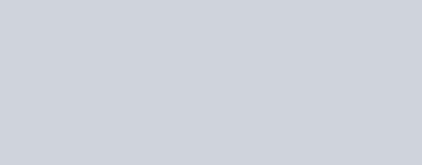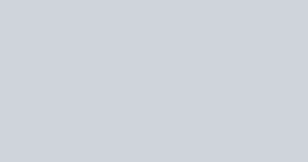A conducting bar of length L is free to slide on two parallel conducting rails as shown in the figure
Question:

A conducting bar of length L is free to slide on two parallel conducting rails as shown in the figureTwo resistors $R_{1}$ and $R_{2}$ are connected across the ends of the rails. There is a uniform magnetic field $\vec{B}$ pointing into the page. An external agent pulls the bar to the left at a constant speed $v$.

The correct statement about the directions of induced currents $I_{1}$ and $I_{2}$ flowing through $R_{1}$ and $\mathrm{R}_{2}$ respectively is :

1. Both $\mathrm{I}_{1}$ and $\mathrm{I}_{2}$ are in anticlockwise direction

2. Both $\mathrm{I}_{1}$ and $\mathrm{I}_{2}$ are in clockwise direction

3. $\mathrm{I}_{1}$ is in clockwise direction and $\mathrm{I}_{2}$ is in anticlockwise direction

4. $I_{1}$ is in anticlockwise direction and $I_{2}$ is in clockwise direction

Correct Option: , 3

Solution: